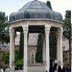# Have a great and prime 2011So think of this: every January 1 is the start of a new year, but “2011” is rather unique. It will start with the combo 1/1/11. 2011 is also the sum of 3 consecutive prime numbers: 2011 = 661 + 673 + 677, the sum of whose digits equals “49”, i.e., 7 (a prime number) squared, also 3 itself is a prime number and so, 7+3=10. Thus:

Starting with 1/1/11
After 10 days 1/11/11;
After 10 months 11/1/11;
After 10 months and 10 days 11/11/11.

Is this coincidence or what?

For the more mathematically inclined (e.g., number theorists), 2011 is also a prime number that is the sum of eleven (11)!! consecutive prime numbers: 2011=157+163+167+173+179+181+191+193+197+199+211

The date 1/1/11 will be repeated in 100 years but 2011 will never happen again!!

So have a great and prime 2011!!